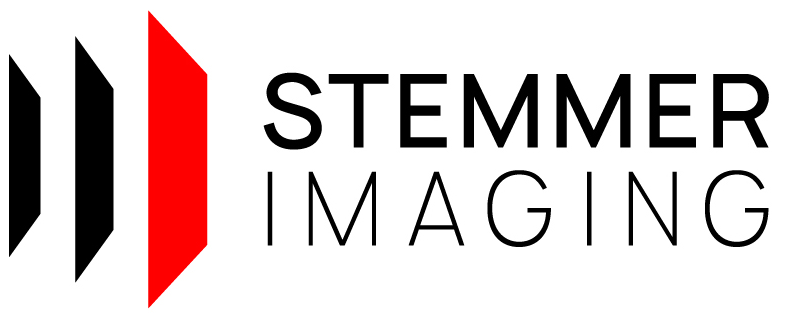CVB++ 14.0
Foundation/CppMetricCalibration
1// ---------------------------------------------------------------------------
17// ---------------------------------------------------------------------------
18
19
20#include <iostream>
21
22#include "cvb/point_cloud_factory.hpp"
23#include "cvb/calibrator_3d.hpp"
24#include "cvb/foundation/metric_aqs12.hpp"
25
26
28void PrintTrafo(const Cvb::AffineMatrix3D& trafo);
29void PrintResiduals(const std::array<Cvb::Point3D<double>, 12>& residuals);
30void PrintAqs12Points(const std::vector< Cvb::Point3D<double>>& points);
31bool CheckAccuracy(const std::array<Cvb::Point3D<double>, 12>& residuals, double desiredAccuracy);
32
33int main()
34{
35 try
36 {
37 std::cout << "Estimation of homography and affine transformation (correcting an inclined laser plane)\n\n";
38
39 // load range map of AQS12
40 const auto rangemapFile = Cvb::InstallPath() + CVB_LIT("tutorial/Metric/Images/RangeMapCalibrationPattern.tif");
42 std::cout << "Rangemap loaded with size of " << rangemap->Width() << " x " << rangemap->Height() << " from " << rangemapFile << ".\n\n";
43
44 // create calibration configuration object
45 auto aqs12 = GetAqs12();
47
48 // create AQS12 segmentor for range maps
50
51 // estimate calibration parameters
54 std::tie(calibrator, residuals) = Cvb::Foundation::Metric::CreateCalibratorFromAqs12Piece(rangemap->Plane(0), *segmentor, *config);
55
56 // show results
57 if(auto trafo = calibrator->CorrectionOfLaserPlaneInclination())
58 PrintTrafo(*trafo);
59 PrintResiduals(residuals);
60
61 // check intermediate results if residuals are not ok
62 double desiredAccuracy = 0.05;
63 if (!CheckAccuracy(residuals, desiredAccuracy))
64 {
65 std::cout << "Results do not have desired accuracy. Check face segmentation and extracted AQS12 points...\n";
66 auto facesAqs12 = segmentor->FaceSegmentationFromPiece(rangemap->Plane(0));
67 auto pointsAqs12 = segmentor->ExtractProjectedPointsFromPiece(rangemap->Plane(0));
68 PrintAqs12Points(pointsAqs12);
69 }
70 else
71 {
72 // create calibrated cloud
73 auto calibratedCloud = Cvb::PointCloudFactory::Create<Cvb::DensePointCloud>(rangemap->Plane(0), *calibrator, Cvb::PointCloudFlags::Float | Cvb::PointCloudFlags::XYZConfidence);
74
75 std::cout << "The calibration was sucessful and accuracy is < " << desiredAccuracy << " mm. :)\n";
76 std::cout << "Lattice size of calibrated cloud: " << calibratedCloud->LatticeSize().Width() << " x " << calibratedCloud->LatticeSize().Height() << "\n";
77 }
78 }
79 catch (const std::exception & error)
80 {
81 std::cout << error.what() << std::endl;
82 }
83
84 return 0;
85}
86
88{
89 // reference points in mm in a right-handed coordindate system
91 { {
92 {20.0018, 44.9941, 15.0000},
93 {24.0018, 39.9942, 14.9994},
94 {23.9994, 24.9972, 15.0001},
95 {20.0021, 20.0035, 15.0011},
96 {15.9994, 25.0079, 15.0016},
97 {16.0000, 39.9919, 15.0010},
98 {20.0095, 59.9985, 4.9902},
99 {32.0093, 44.9958, 4.9909},
100 {32.0052, 19.9925, 4.9920},
101 {20.0021, 4.9961, 4.9939},
102 { 8.0024, 19.9980, 5.0009},
103 { 8.0065, 45.0009, 4.9984},
104 } };
105
107}
108
109bool CheckAccuracy(const std::array<Cvb::Point3D<double>, 12>& residuals, double desiredAccuracy)
110{
111 for (auto residual : residuals)
112 {
113 if (std::abs(residual.X()) > desiredAccuracy || std::abs(residual.Y()) > desiredAccuracy || std::abs(residual.Z()) > desiredAccuracy)
114 {
115 return false;
116 }
117 }
118 return true;
119}
120
121void PrintTrafo(const Cvb::AffineMatrix3D& trafo)
122{
123 std::cout << "Estimated transformation:\n";
124 std::cout << "translation: [";
125 std::cout << trafo.Translation().X() << ", " << trafo.Translation().Y() << ", " << trafo.Translation().Z() << "]\n";
126
127 std::cout << "transformation matrix: [\n";
128 std::cout << trafo.Matrix() << ", " << trafo.Matrix() << ", " << trafo.Matrix() << ",\n";
129 std::cout << trafo.Matrix() << ", " << trafo.Matrix() << ", " << trafo.Matrix() << ",\n";
130 std::cout << trafo.Matrix() << ", " << trafo.Matrix() << ", " << trafo.Matrix() << "]\n\n";
131}
132
133void PrintResiduals(const std::array<Cvb::Point3D<double>, 12>& residuals)
134{
135 std::cout << "Residuals:\n";
136 for (auto residual : residuals)
137 {
138 std::cout << residual.X() << " " << residual.Y() << " " << residual.Z() << "\n";
139 }
140 std::cout << "\n";
141}
142
143void PrintAqs12Points(const std::vector< Cvb::Point3D<double>>& points)
144{
145 std::cout << "AQS12 points\n";
146 for (auto point : points)
147 {
148 std::cout << point.X() << " " << point.Y() << " " << point.Z() << "\n";
149 }
150 std::cout << "\n";
151}
Affine transformation matrix for 3D.
Definition: affine_matrix_3d.hpp:98
Vector3D< double > Translation() const noexcept
Gets the translation part of the transformation.
Definition: affine_matrix_3d.hpp:157
Matrix3D Matrix() const noexcept
Gets the matrix part of the transformation.
Definition: affine_matrix_3d.hpp:137
Object to collect all input parameters for the AQS12 calibration piece.
Definition: decl_metric_aqs12_calibration_piece.hpp:28
static std::shared_ptr< AQS12RangeMapSegmentor > Create(const SegmentationMethod method)
Creates a AQS12 segmentor for range maps based on given segmentation method.
Definition: decl_metric_segmentor_range_map_aqs12.hpp:35
static std::unique_ptr< CalibrationConfiguration > Create(const AQS12Piece &aqs12)
Creates a calibration configuration object.
Definition: decl_metric_calibration_configuration.hpp:51
static std::unique_ptr< Image > Load(const String &fileName)
Loads an image with the given file name.
Definition: detail_image.hpp:32
@ KmeansClustering
Clustering top, base and faces using kmeans.
AQS12CalibratorResult CreateCalibratorFromAqs12Piece(const ImagePlane &imagePlane, const AQS12RangeMapSegmentor &segmentor, const CalibrationConfiguration &config)
Calculates intrinsic calibration parameters from the given range map image of an AQS12 calibration pi...
Definition: metric_aqs12.hpp:68